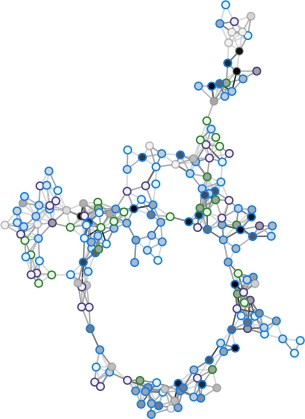Queueing-tool: A network simulator¶

Queueing-tool is a simple agent based network simulator. The queues are used to add congestion between nodes.

Getting set up is simple:

>>> import queueing_tool as qt
>>> import networkx as nx
>>> g = qt.generate_random_graph(200, seed=3)
>>> q = qt.QueueNetwork(g, seed=3)
>>> q.max_agents = 20000
>>> q.initialize(100)
>>> q.simulate(10000)
...
>>> pos = nx.nx_agraph.graphviz_layout(g.to_undirected(), prog='fdp')
>>> scatter_kwargs = {'s': 30}
>>> q.draw(pos=pos, scatter_kwargs=scatter_kwargs, bgcolor=[0,0,0,0],
...        figsize=(10, 16), fname='fig.png',
...        bbox_inches='tight')